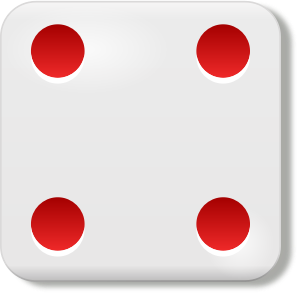# Probability distribution

## In words...

The probability distribution of a random variable encodes the statistical behavior of the random variable. More precisely, it is a function that computes the probability of the random variable taking a value in a particular set for all possible sets of values.

A probability distribution is defined from the probability measure associated to the considered experiment, which measures the probability of the outcomes of this experiment.

## In picture...

### A discrete example: the roll of a die

In the roll of a die, where the outcome is the face on top of the die when it stops, the sample space is the set of all these faces: $\Omega = \left\{ \right.$,,,,,$\left.\right\}$
and we can define the random variable $X : \Omega \rightarrow \{1,2,3,4,5,6\}$ that returns the number of dots on the face on top of the die.

### Example of a continuous random variable

Here is a population of individuals of various ages.
We consider a random experiment in which we pick one individual at random from the population and we let the random variable $X$ be his age (in years).
Depending on the probability of picking individuals and their age, we observe the values of $X$ with different probabilities.

$X =$

## In maths...

### Probability distribution of a discrete random variable

The probability distribution of a discrete random variable $Y$ defined on the probability space $(\Omega, \Sigma, P)$ and taking values in $\Y$ is defined via the probability mass function $p_Y : \Y \rightarrow [0,1]$, which is the function that yields the probability of observing any value $y$ of $\Y$: $$p_Y(y) = P(Y = y) = P( \{ \omega\in \Omega\ :\ Y(\omega) = y\} ) .$$ The probability distribution of $Y$ is the probability measure $P_Y : \mathcal{B}(\Y) \rightarrow [0,1]$ defined as $$P_Y( A ) = \sum_{y\in A} P(Y = y )$$ for the probability space $(\Y, \mathcal{B}(\Y), P_Y)$, where $\mathcal{B}(\Y)$ denotes a $\sigma$-algebra over $\Y$.

### Probability distribution of a continuous random variable

The probability distribution of a continuous random variable $X$ defined on the probability space $(\Omega, \Sigma, P)$ and taking values in $\R$ is the proability measure $P_X : \mathcal{B}(\R) \rightarrow [0,1]$ defined as $$P_X( A ) = P( X \in A ) = P( \{ \omega\in \Omega\ :\ X(\omega) \in A \}$$ for the probability space $(\R, \mathcal{B}(\R), P_X)$, where $\mathcal{B}(\R)$ denotes a $\sigma$-algebra over the real numbers $\R$.

The probability distribution of a continuous random variable can be computed from its probability density function.